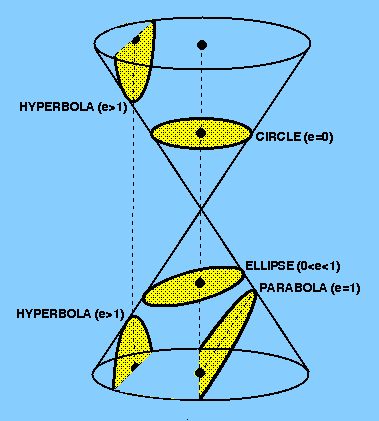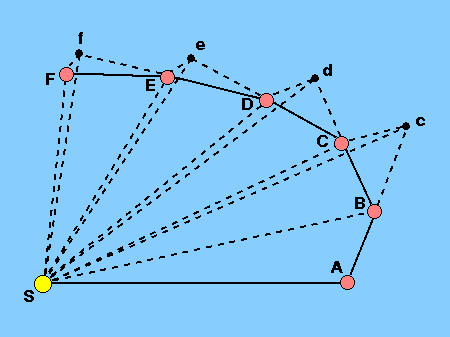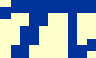All three of Kepler's laws follow from Newton's laws of motion when the law of universal gravitation is used to express the forces between the Sun and the planets.

kepler I      Newton's derivation of Kepler's first law is embodied in his statement and solution of the so-called two-body problem.

Given at any time the positions and velocities of two massive particles moving under their mutual gravitational force, the masses also being known, provide a means of calculating their positions and velocities for any other time, past or future.

The solution of the two-body problem is an equation of motion. Its derivation is outside the scope of this course, as it requires the use of vector calculus in conjunction with Newton's second law and his law of gravitation. The solution for two masses m1 and m2 can be written in polar coordinates r,(see Figure 31) as follows:

r = h2 / G(m1+m2) (1 + e cos),

where h is a constant which is twice the rate of description of area by the radius vector and e is the eccentricity of the orbit. This equation looks similar to the polar equation of an ellipse that we derived earlier. In fact, it is the polar equation of a conic section.

The ellipse is just one example of a class of curves called conic sections, which are formed when a cone is cut with a plane, as shown in Figure 35. When the plane is perpendicular to the cone's axis, the result is a circle (ellipticity, e = 0); when it is parallel to one side, the result is a parabola (e = 1); intermediate angles result in ellipses (0 < e < 1). A hyperbola results when the angle the plane makes with the cone's side is greater than the opening angle of the cone (e > 1).

 figure 35: Conic sections.In obtaining his solution to the two-body problem, Newton generalized Kepler's first law. He deduced that when one body moves under the gravitational influence of another, the orbit of the moving body must be a conic section. Planets, satellites and asteroids have elliptical orbits. Many comets have eccentricities so close to unity that they follow essentially parabolic orbits. A few comets have hyperbolic orbits - after one perihelion passage, such comets leave the solar system forever. Space probes have been launched into hyperbolic orbits with respect to the Earth, but they are nearly always captured into elliptical orbits about the Sun. Pioneer 10 was the first spacecraft that, when perturbed by Jupiter, escaped from the solar system.

kepler II      There are two ways in which it is possible to derive Kepler's second law from Newton's laws. The first, presented by Newton in 1684, is a geometrical method and is shown in Figure 36.

 figure 36: Newton's proof of Kepler's second law.Newton visualized the motion of an object acted on by a gravitational force as a succession of small kicks or impulses which in the limit become a continuously applied influence. Newton imagined an object travelling along part of an orbit AB which then receives an impulse directed towards the point S. As a result, it then travels along the line BC instead of Bc. Similar impulses carry it to D, E and F. Newton visalized the displacement BC as being, in effect, the combination of the displacement Bc, equal to AB, that the object would have undergone if it had continued for an equal length of time with its original velocity, together with the displacement cC parallel to the line BS along which the impulse was applied. This at once yields Kepler's second law by a simple argument: The triangles SAB and SBc are equal, having equal bases (AB and Bc) and the same altitude. The triangles SBc and SBC are equal, having a common base (SB) and lying between the same parallels. Hence triangle SAB = triangle SBC.

A modern Newtonian derivation of Kepler's second law requires the concept of an orbiting body's angular momentum

L = r X p = m (r X v)

where m is the body's mass, r is its position vector and p its linear momentum (= mv, where v is its velocity). Note that for the first time in this course we distinguish between vector quantities and scalar quantities by writing vector quantities in a bold face. The vector cross product (denoted by X) is an operation that yields the product of the perpendicular components of two vectors; hence if r and p are parallel, then r X p = 0. Angular momentum is a vector quantity L with the units kgm2s-1. Differentiating L, we have

dL/dt = d(r X p)/dt = v X p + r X (dp/dt) = r X F

since v is parallel to p and dp/dt is the definition of force according to Newton's second law. We call dL/dt the torque (with units kgm2s-2) and see that when F and r are co-linear, due to a central force such as gravitation, the torque vanishes. Hence L is constant in time and so angular momentum is conserved for all central forces. The conservation of angular momentum is a very powerful tool in celestial mechanics and can be used to derive Kepler's second law as follows.

 figure 37: The velocity components of a body in an elliptical orbit.A body is moving in an elliptical orbit with a velocity v at a distance r from the focus F (Figure 37). During a short time intervalt, the body moves from P to Q and the radius vector sweeps through the angle. This small angle is approximately given by= vtt / r, where vt is the component of v perpendicular to r. During this time, the radius vector has swept out the triangle FPQ, the area of which is approximately given byA = rvtt / 2. Therefore, in the limit given byt approaching zero, we have

dA/dt = rvt/2 = ½r2(d/dt).

Now, the angular momentum of the body in Figure 37 is given by the vector perpendicular to the plane defined by r and v, i.e. it is out of the plane of the paper. The scalar magnitude of L is given by

L = mvtr= mr2 d/dt.

This means that the rate of sweeping out area is given by

dA/dt = ½r2(d/dt) = L / 2m.

As L and m are constants, then dA/dt must be a constant, i.e. the rate of sweeping out area is a constant. Hence we have verified Kepler's second law.

kepler III      Newton's form of Kepler's third law can be derived by considering two bodies of masses m1 and m2, orbiting their (stationary) centre of mass at distances r1 and r2 (Figure 38).

 figure 38: Two bodies in orbit about their common centre of mass.Because the gravitational force acts only along the line joining the centres of the bodies, both bodies must complete one orbit in the same period P (though they move at different speeds v1 and v2). The forces on each body due to their centripetal accelerations are therefore

F1 = m1v12 / r1 = 42 m1r1 / P2
F2 = m2v22 / r2 = 42 m2r2 / P2.

Newton's third law tells us that F1 = F2, and so we obtain

r1 / r2 = m2 / m1.

This tells us that the more massive body orbits closer to the centre of mass than the less massive body. The total separation of the two bodies is given by

a = r1 + r2

which gives

r1 = m2a / (m1 + m2).

Combining this equation with the equation for F1 derived above and Newton's law of gravitation (Fgrav = F1 = F2 = Gm1m2 / a2) gives Newton's form of Kepler's third law:

P2 = 42 a3 / G(m1 + m2).

If body 1 is the Sun and body 2 any planet, then m1 >> m2. Hence the constant of proportionality in Kepler's third law becomes 42 / GMSun.